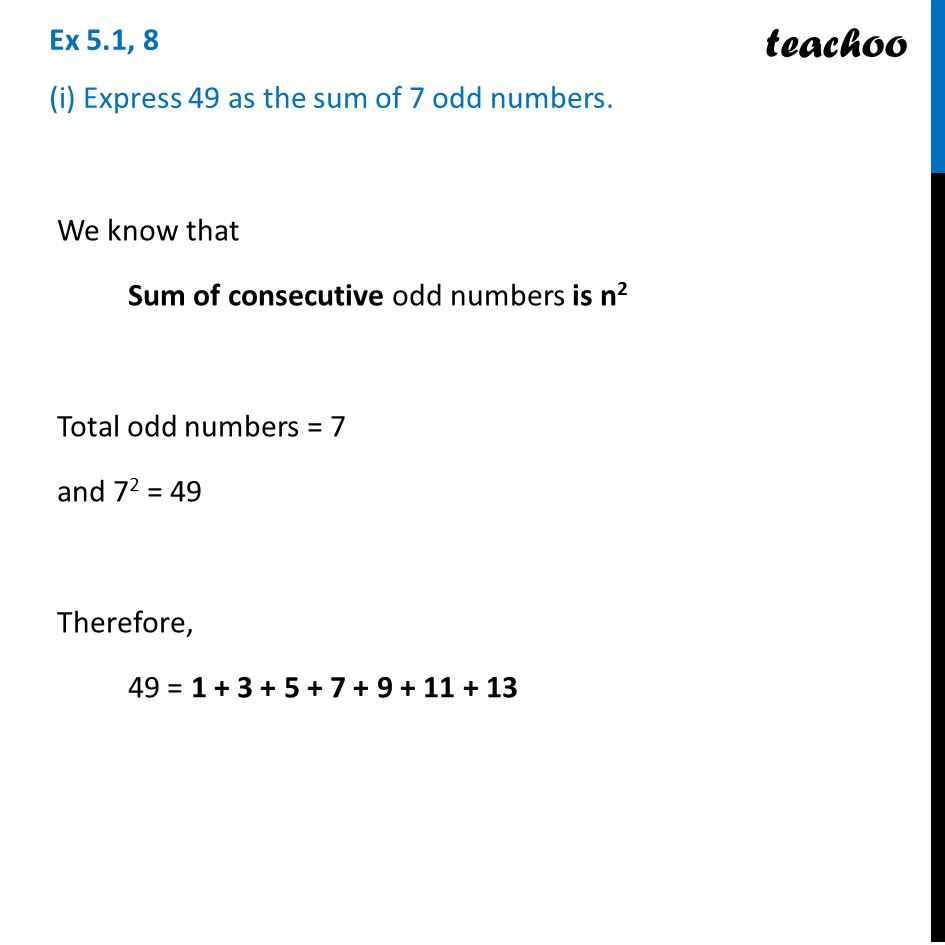Sum of consecutive odd numbers

Chapter 5 Class 8 Squares and Square Roots
Concept wiseLearn in your speed, with individual attention - Teachoo Maths 1-on-1 Class

### Transcript

Ex 5.1, 8 (i) Express 49 as the sum of 7 odd numbers.We know that Sum of consecutive odd numbers is n2 Total odd numbers = 7 and 72 = 49 Therefore, 49 = 1 + 3 + 5 + 7 + 9 + 11 + 13